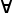Maths - Proof

On these pages we look at how to do mathematical proofs and also the extent that computers can be used in proving things.

Proofs

A proof allows us to start with known information and to derive other information from it. So proofs don't create information from nothing, they are more like:

if some set of facts then some other thing

The starting information for a proof may be the results of other proofs or it may be the things that we assume to be true (known as axioms or postulates). So, ultimately, everything derives from a set of postulates, the things we assume. For a given mathematical structure it is not always clear what the postulates should be, it may be that:

we assume A and prove B from it.

or it may be that:

we assume B and prove A from it.

In some cases it could be that neither A or B is more fundamental than the other, it may come down to an arbitrary decision about which we choose to be the postulate.

In addition to the mathematical system that we are analysing we also need a system of logic a set of rules to derive things from other things. In this sense logic is used as a meta-mathematics operating on other mathematics.

Mathematical Proofs and Computers

Introduction
COQ   coq
Isabelle

On these pages have included some code for the program Isabelle which is a proof assistant. I have put some information about how I installed this program on this page.

Many of the important results in mathematics have proofs that can be computerised.

Terminology

Notation Meaning
p ⇒ q infer (If p, then q)

Direct Proof

Prove a statement by a combination of established facts (existing lemmas and theorems)

Common proof rules used are modus ponens and universal instantiation.

Universal Instantiation

Example: "All men are mortal, Socrates is a man, therefore Socrates is a mortal."x A(x) ⇒ A(a/x)

Nonconstructive Proof

 metadata block see also: Correspondence about this page Book Shop - Further reading. Where I can, I have put links to Amazon for books that are relevant to the subject, click on the appropriate country flag to get more details of the book or to buy it from them.Modern Graph Theory (Graduate Texts in Mathematics, 184) Commercial Software Shop Where I can, I have put links to Amazon for commercial software, not directly related to the software project, but related to the subject being discussed, click on the appropriate country flag to get more details of the software or to buy it from them.

This site may have errors. Don't use for critical systems.